# Catch up on the latest AI articles# How Can The Fine-tuning Instability Of BERT Be Resolved?

3 main points
✔️ Analyze instability of fine-tuning of transformer-based pre-training models such as BERT
✔️ Identify initial optimization difficulties due to gradient vanishing and differences in generalization as sources of instability
✔️ Proposed a new baseline to improve the stability of fine-tuning

On the Stability of Fine-tuning BERT: Misconceptions, Explanations, and Strong Baselines
written by Marius MosbachMaksym AndriushchenkoDietrich Klakow
(Submitted on 8 Jun 2020 (v1), last revised 6 Oct 2020 (this version, v2))

Subjects: Machine Learning (cs.LG); Machine Learning (stat.ML)

## first of all

Transformer-based pre-training models, such as BERT, have been shown to perform well on a variety of tasks with fine-tuning. In contrast to such good performance, BERT's fine-tuning is unstable. That is, depending on the various random seeds, the performance of the task can vary significantly.

Catastrophic forgetting and small dataset size have been hypothesized as reasons for such instability of fine-tuning. In the paper presented in this article, we show that these hypotheses cannot explain the instability of fine-tuning.

Furthermore, we analyzed BERT, RoBERTa, and ALBERT and showed that the instability of fine-tuning is caused by two aspects: optimization and generalization. In addition, based on the analysis results, we proposed a new baseline that can perform fine-tuning in a stable manner.

## experiment

### data set

For the analysis of fine-tuning, we use the four datasets shown below.

The statistics of the data set are as follows.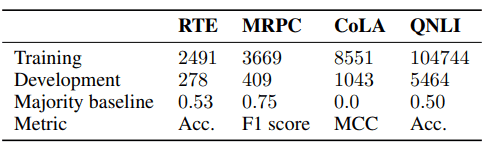These are all benchmarks that are included in the GLUE task. Of the four, previous work has shown that CoLA is particularly stable for fine-tuning and RTE is particularly unstable.

The setting of hyperparameters, etc.

The settings of hyperparameters and models for fine-tuning are as follows.

• Model: uncased BERT-LARGE (or RoBERTa-LARGE, ALBERT-LARGE)
• Batch size: 16
• Learning rate: 2e-5 (increases linearly from 0 to 2e-5 in the first 10% of iterations, then decreases linearly to 0)
• Dropout rate:$p=0.1$(0 in ALBERT)
• weight decay:$\lambda=0.01$(0.1 in RoBERTa, no gradient clipping)

The LARGE model is used because it does not cause instability during fine-tuning of BERT-BASE.

The stability of fine-tuning is judged based on the magnitude of the standard deviation of the performance (F1 score, accuracy, etc.) during fine-tuning against the randomness of the algorithm.

About the failure judgment of the execution

If the accuracy at the end of the training is less than or equal to the accuracy of many classifiers corresponding to each dataset, the fine-tuning is judged as "failed".

## On the hypothesis of the cause of fine-tuning instability

Previous studies have hypothesized catastrophic forgetting and small data set size as reasons for the instability of fine-tuning.

In the experiment, we first test these hypotheses.

Does catastrophic forgetting induce fine-tuning instability?

Catastrophic forgetting refers to the phenomenon where the performance of a trained model on a previous task degrades when it is trained on another task. In the setting of this experiment, this corresponds to the inability to properly execute tasks (such as MLM) during pre-training, such as BERT, after fine-tuning.

To investigate the relationship between this catastrophic forgetting and instability, we conduct the following experiment.

• Perform fine-tuning of BERT on RTE data sets.
• Select three successful training trials and three unsuccessful trials respectively.
• For them, we measure and evaluate the perplexity of MLM (Masked Language Modeling) based on the test set of the WikiText-2 language modeling benchmark.
• We investigate the relationship between catastrophic forgetting and instability by replacing the upper $k$ layers ($0 \leq k \leq 24$) of the 24 layers with pre-trained models. (When $k=0$, all the layers are fine-tuned models, and when $k=24$, all the layers are pre-trained models.)

The results of this experiment are as follows.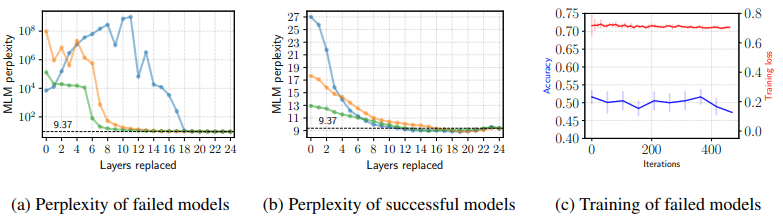In the failed model (left panel), we can see that catastrophic forgetting does indeed occur (note the values on the vertical axis). When we replace the top 10 layers except for the light blue layer, the perplexity improves, suggesting that the top 10 layers are mainly affected by catastrophic forgetting. In addition, catastrophic forgetting should usually be caused by adaptation to a new task.

However, when fine-tuning fails, the training loss is somewhat small ($\simeq -ln(\frac{1}{2})$), although the accuracy is low (right panel). It is pointed out in the paper that this suggests that fine-tuning failures are caused by optimization problems.

Does the smallness of the data set cause fine-tuning instability?

Next, to investigate the relationship between dataset size and fine-tuning instability, we perform the following experiments.

• We randomly sample 1000 cases from the CoLA, MRPC, and QNLI training sets.
• We perform fine-tuning of BERT with different random seeds 25 times for each data set.
• We experiment with the same number of epochs as usual (3 epochs) and the same number of iterations as usual and compare the results in each setting.

The results at this time are as follows.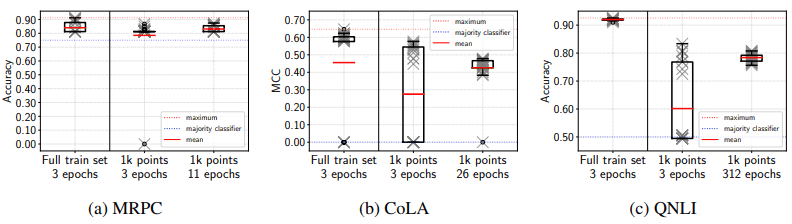As shown in the figure, when we train the same number of epochs as usual (1k points 3 epochs), CoLA and QNLI fail more often and the variance is larger. However, when the number of iterations is the same as usual, the variance is sufficiently small. Also, the number of failed trials is reduced to 0 for MRPC and QNLI, and 1 for CoLA, showing the same stability as when training on the complete training set.

This suggests that the instability of fine-tuning is caused by the insufficient number of iterations, rather than the insufficient training data size.

## What is the cause of the instability of fine-tuning?

Our experiments have shown that catastrophic forgetting and data set size are correlated with fine-tuning instability, but they are not the main causes of it. In the following sections, we will further explore what causes instability in fine-tuning.

Specifically, we investigate (i) optimization and (ii) generalization.

### (i) Optimization.

If fine-tuning fails, the following shows that gradient loss occurs.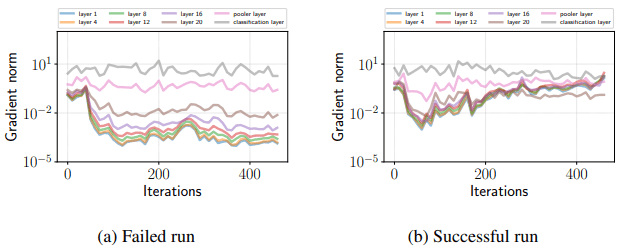As shown in the figure, in the unsuccessful trials, the gradient becomes very small in the early stage of training except for the classification layer, and it does not return to the original state. On the other hand, in the successful trials, the gradient becomes small in the first 70 iterations, but it becomes large after that, and no gradient loss occurs.

In the case of RoBERTa and ALBERT, the same gradient loss occurs as shown in the following figure.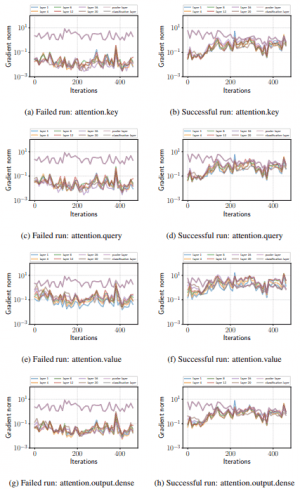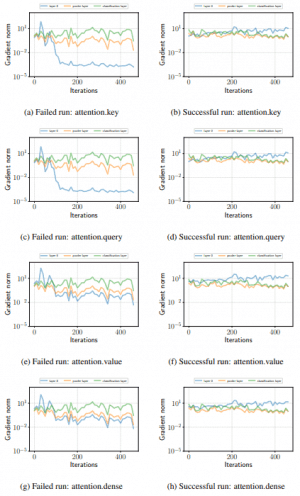(Left: RoBERTa, Right: ALBERT)

Thus, we can expect that the instability of fine-tuning is strongly related to optimization failures such as gradient disappearance.

The results of using the ADAM bias correction during fine-tuning of BERT are shown below.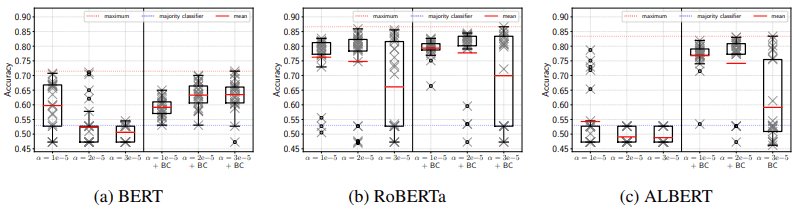The $\alpha$ indicates the training rate and BC indicates the Bias Correction.

As shown in the figure, we can see that a large performance improvement occurs for BERT and ALBERT, and some improvement is also observed for RoBERTa. Thus, the bias correction of ADAM leads to a performance improvement during fine-tuning.

Visualization of loss surface

A visualization of the loss during fine-tuning on a two-dimensional surface is shown below.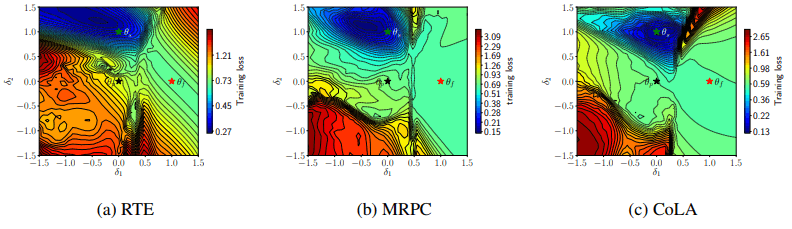The $\theta_p,\theta_f,\theta_s$ in the figure represent the pre-training model, the model that failed fine-tuning, and the model that succeeded, respectively. The lines represent contour (equilibrium) lines. The figure shows that the failed model is located in the region with small loss (blue area) and converges to a very different location (like a deep valley) from the successful and pre-training models.

The visualization of the gradient norm is also shown below.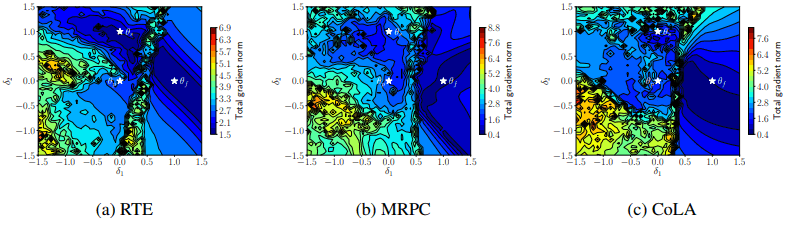At this point, there is a mountainous barrier of dense contour lines between the failed model $\theta_f$ and the others, and these are now separated (this is the case for all three datasets).

From these results (confirmation of gradient vanishing, performance improvement due to ADAM bias correction, and qualitative analysis from loss and gradient visualization), we can predict that the fine-tuning instability may be due to an optimization failure.

### (ii) On generalization.

Next, we focus on the relation between fine-tuning instability and generalization.

First, we show below the accuracy for the development set of successful trials (10) for the case of fine-tuning of BERT on RTE.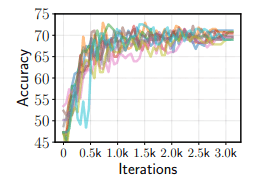In addition, for the results of 450 trials performed in different settings, the accuracy, and training loss for the DEVELOPMENT set are as follows.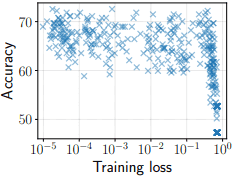In this figure, the accuracy is almost unchanged in the range of training loss from $10^{-5}$ to $10^{-1}$. In other words, overfitting (overfitting to the training data) does not occur during fine-tuning. Therefore, even if we train until the training loss becomes very small (with a large number of iterations), we can expect that the performance degradation due to overfitting will not be a problem.

## A simple baseline for stable fine-tuning

Given the research on the instability of fine-tuning and its relation to optimization and generalization, a simple strategy to increase stability (on small datasets) would be to

• To avoid gradient loss in the early stages of training, we use a small learning rate with bias correction.
• Increase the number of iterations significantly and train for nearly zero training loss

In accordance with these, a simple baseline was proposed as follows

• Using ADAM with bias correction and learning rate set to 2e-5
• The training was performed for 20 epochs, and the learning rate increased linearly for the first 10% and decayed to zero thereafter
• Other hyperparameters are not changed.

## result

Based on the above baseline, the results of the run are as follows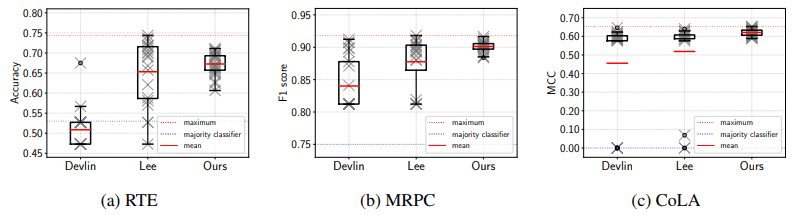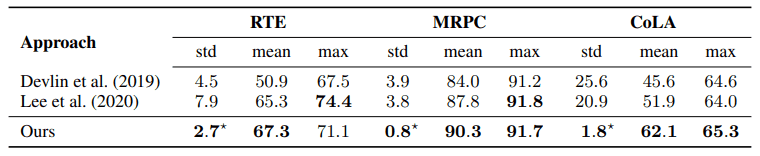It is shown that the proposed method yields more stable fine-tuning in terms of final performance and standard deviation compared to the default settings proposed in the BERT paper and Mixout, a recent improvement method.

## summary

In the paper presented in this article, we have shown that the instability of fine-tuning in BERT is due to two aspects: optimization and generalization. Furthermore, based on the results of these analyses, we proposed a simple baseline that stabilizes fine-tuning.

BERT and other Transformer-based pre-training models have shown very good performance. Improving the fine-tuning stability of these models is a serious challenge. Therefore, this study is very important because it analyzes the causes of fine-tuning instability and shows an effective method to stabilize the training.

If you have any suggestions for improvement of the content of the article,